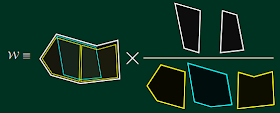## Thursday, March 14, 2013

### Pentagon transitions: tools to solve planar $$\NNN=4$$ at finite coupling

Benjamin Basso, Amit Sever, and Pedro Vieira have released an interesting preprint:
Space-time S-matrix and Flux-tube S-matrix at Finite Coupling (PDF)
Note that if you download it in other formats by clicking at "Download Source" which you may open in WinRAR etc., you will acquire an additional TXT file with some bonus formulae.A typical schematic expression for a Wilson loop vev, in terms of ratios of expressions denoted by squares and pentagons.

They propose a way to calculate the scattering amplitudes in the maximally supersymmetric, $$\NNN=4$$ gauge theory in $$d=4$$, without any approximations, using funny pictures with lots of pentagons!

Note that the gauge theory in $$d=4$$ may be viewed as an ordinary quantum field theory with a perturbative expansion given by the Feynman diagrams.

Already in the 1970s, Gerardus 't Hooft ingeniously realized that in the limit of many colors, $$N_c\to\infty$$, the Feynman diagrams begin to fill spider nets – two-dimensional areas which look like and actually are string world sheets. Their topology matters (less complicated topologies are dominant) and may be reinterpreted as the topology of a string diagram which could have indeed be identified with the stringy expansion of type IIB string theory on an AdS space (with a previously unexpected extra dimensions) in the wake of Maldacena's 1997 AdS/CFT breakthrough.

The stringy interactions add "handles" to the world sheet – their contributions are suppressed by $$g_s\sim g_{YM}^2\sim 1/N_c\ll 1$$ if the 't Hooft coupling $$\lambda=g_sN_c$$ is kept fixed. But already at the leading, handle-free level, the so-called "planar limit" (tree-level string theory), the theory is interesting. The theory living on the planar stringy world sheet is interacting (not free) and non-trivial. On the other hand, it has so many symmetries – such as the dual superconformal symmetry and the Yangian symmetry that it generates together with the ordinary superconformal symmetry – that the theory is basically solvable (we say "integrable").

To write down explicit solutions to the questions about the gauge theory in its planar limit is a hard enough but not hopeless task and the discovery of the full formulae has been a holy grail of many brilliant physicists.

In the recent 10 years, various methods to solve it have been improved. In most cases, one converted the gauge theory to a perturbative expansion at the strong coupling – the string theory in the AdS space – or a perturbative expansion of the field theory at the weak coupling which could have been organized in new ways (e.g. by the methods of the twistor minirevolution).

This new paper is a step in a completely new journey – started 3 years ago or so by some of the authors and by Alday, Gaiotto, and Maldacena – to approach these questions.

The task they claim to have solved is to calculate the scattering amplitudes of $$n$$ gluons or other "elementary excitations" in the planar limit. Note that this limit means that we neglect the subleading $$1/N_c\sim g_s$$ corrections but we are interested in the dependence of the result on $$\lambda\sim g_s N_c$$, the 't Hooft coupling. This task is equivalent to the computation of the expectation value of a Wilson loop but the Wilson loop must have the simple shape of a polygon with $$n$$ vertices corresponding to the gluons.

Their recipe is to divide the polygon to a tree of $$n-4$$ mutually overlapping pentagons so that adjacent pentagons have $$n-5$$ tetragons (squares) as their intersections. To a large extent, the squares are "trivial" and resemble two-point functions in ordinary string theory. The pentagons are the elementary interactions and resemble the three-point functions in textbook string theory. Hexagons are still somewhat special and heptagons (with 7 vertices) are the first generic polygons.

They associate some mathematical objects with these pentagons, especially "pentagon transitions" which is a special name for pentagons with two marked points (similar to vertex operator insertions in ordinary textbook string theory). They associate some mathematical objects to these pentagons and impose some axioms.

After some work, they're capable of computing the scattering amplitude "exactly" (in principle) by solving some equations. Their strategy may be viewed as an example of the bootstrap paradigm. Regular TRF readers know that I have often mentioned the bootstrap approach as a black horse of string theory that was standing by string theory's cradle, got largely abandoned, but that may allow us – sometime in the future – to define and calculate string theory at generic points of the "landscape", in the middle, without approximations. If that's the case, such a bootstrap approach may become fundamental again.

These three physicists' paper is a particular realization of my vision, albeit in the limited context of planar gauge theory which means type IIB string theory in an AdS background with stringy loops neglected. Such a calculation at a finite $$\lambda$$ is possible because the dynamics of the Gubser-Klebanov-Polyakov flux tube (linked to the tetragons i.e. squares in the geometric picture of this paper) is fully integrable.

Many of their tricks and axioms are similar to those that are employed by Nima Arkani-Hamed and collaborators but they look technically inequivalent. It remains to be seen whether some deeper unification of these approaches exists and how far these approaches may be generalized in our efforts to fully define and perhaps solve quantum field theory and string theory "everywhere".

1.This was intriguing and rather exiting reading to me (or even to someone as much on the surface of this topic as I)!
You, Lubos, and your communications are a treasure, FOR REAL!!!
P

2.Clearly you have not been following high energy physics in the last 3 years if you think this idea is new ;0

3.Yeah, I enjoy reading such articles (I mean the TRF post not the original awesome paper ...:-P) a lot too. It is so nice of Lumo explaining these things such that everybody can take something out of it :-)

Cheers